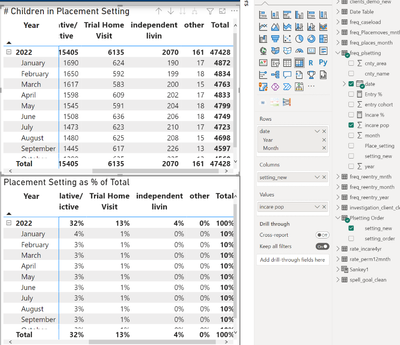cancel
Showing results for
Did you mean:Helper I

## Create Dax Measure to show column category values as Percentages of Row Total in Matrix Table

Hi All,

I am creating a Metrix visual from a data table that shows the date and daily count of children in different type of foster care placement (3 columns/fields: date, count, placement type). In the screenshot of Metrix visuals below, the top one is the count (sum) of children by year/month and placement type. The bottom one is to show the count as percentage of row totals. Since the Power BI built-in "show value as % as row totals" have 2 decimal points and cannot  be formatted, I need to create a dax measure to have the % values. I created one based on a posting in this forum which recommends using the formulae:

PERCENT = SUM([TOTAL SALES]) / SUMX(ALL(table),[TOTAL SALES])However, as shown in the screenshot above, the row percentage values are right only for the Year 2022 total, but not for the monthly vlaues. The monthly %s are not row %, but as % of column total. I would like to have a dynamic % that shows all monthly and yearly values as % of row totals. But the formulae I used cannot do this.

Can you suggest any dax formulae that I can use to achieve the task?

Thanks.

2 ACCEPTED SOLUTIONSSuper User

@LijunChen , This will based on grand total

PERCENT = SUM([TOTAL SALES]) / SUMX(ALL(table),[TOTAL SALES])

If you need by year

PERCENT =divide( SUM([TOTAL SALES]) , calculate(SUM([TOTAL SALES]), removefilters(Table[Month]) ) )

You can also use isinscope to get different subtotals

DAX- ISINSCOPE: https://youtu.be/Tu92J_OtUK0

Percent of Total and Percent of Subtotal: https://youtu.be/9JTO1s0KV2UHelper I

Many thanks. I will check this out and see.

2 REPLIES 2Super User

@LijunChen , This will based on grand total

PERCENT = SUM([TOTAL SALES]) / SUMX(ALL(table),[TOTAL SALES])

If you need by year

PERCENT =divide( SUM([TOTAL SALES]) , calculate(SUM([TOTAL SALES]), removefilters(Table[Month]) ) )

You can also use isinscope to get different subtotals

DAX- ISINSCOPE: https://youtu.be/Tu92J_OtUK0

Percent of Total and Percent of Subtotal: https://youtu.be/9JTO1s0KV2UHelper I

Many thanks. I will check this out and see.Announcements#### Exclusive opportunity for Women!

Join us for a free, hands-on Microsoft workshop led by women trainers for women where you will learn how to build a Dashboard in a Day!#### Power Platform Conference-Power BI and Fabric Sessions

Join us Oct 1 - 6 in Las Vegas for the Microsoft Power Platform Conference.Top Solution Authors
Top Kudoed Authors
Users online (1,865)